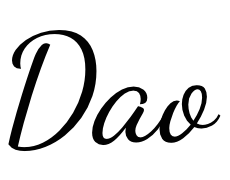# SVG 一览

Jan 10, 2023
#svg

## 引言 #

SVG（Scalable Vector Graphics） 应该是浏览器发展历史以来，曲线绘画支持度最高的一块内容了。HTML 提供了定义段落、表格、标题等内容的元素，SVG 提供了用于定义矩形、复杂曲线图形的元素，之后 SVG 也变得复杂起来，渐变、旋转、动画、滤镜等效果，以及和 JS 交互也都慢慢加上了。

SVG 自 2003 年被 W3C 作为推荐标准以来，最接近的完整版本是 1.1 版，在 2011 年 SVG1.1 的第二个版本成为推荐版本，完整的 SVG1.2 本来是下一个标准版本，但是被 SVG 2.0 取代了。针对移动端，SVG 在 2003 年推出了 SVG TinySVG Basic，在 2008 年 SVG Tiny1.2 成为 W3C 推荐标准。

## 入门 #

SVG 是由 `<svg>` 标签包裹的一组元素，也可以说是 XML 用来描述图形和绘图程序的语言。简单看一个例子：

html
``````<svg
version="1.1"
baseProfile="full"
width="300"
height="200"
xmlns="http://www.w3.org/2000/svg"
>
<rect width="100%" height="100%" fill="rgb(248,113,113)" />
<circle cx="150" cy="100" r="80" fill="rgb(74,222,128)" />
<text x="150" y="125" font-size="60" text-anchor="middle" fill="white">
Dao
</text>
</svg>
``````
``````<svg
version="1.1"
baseProfile="full"
width="300"
height="200"
xmlns="http://www.w3.org/2000/svg"
>
<rect width="100%" height="100%" fill="rgb(248,113,113)" />
<circle cx="150" cy="100" r="80" fill="rgb(74,222,128)" />
<text x="150" y="125" font-size="60" text-anchor="middle" fill="white">
Dao
</text>
</svg>
``````

• version 表示 SVG 遵循的版本规范，只有 `1.0``1.1` 这两个有效选择。
• baseProfile 表示一个 SVG 的描述，取值为 `none` | `full` | `basic` | `tiny`
• widthheight 表示 SVG 整体容器的宽高。
• xmlns 表示 SVG 遵循的命名空间（作为 XML 语言需要正确命名它）。

### 文件属性和类型 #

SVG 中元素的渲染顺序是 “后来居上”，越后面的越可见。在 Web 上渲染 SVG 一般有两种：直接渲染 SVG 文件 和 嵌入到 HTML 中：

• `application/xhtml` 类型的文件，可以直接把 SVG 嵌入 XML 里。
• 可以通过 `<object />``<iframe />``<embed>``<img />``background-image` 以及行内引入，更多的说明可以查看 W3C 对于SVG 使用的说明

SVG 文件同样支持压缩，通过 gzip 的方式压缩之后，会生成 `.svgz` 后缀的压缩文件，当然想要正常访问需要在服务端添加相关 gzip 的配置。对于普通的 SVG 文件，服务端会发送这种请求头：

js
``````Content-Type: image/svg+xml
``````
``````Content-Type: image/svg+xml
``````

js
``````Content-Type: image/svg+xml
Content-Encoding: gzip
``````
``````Content-Type: image/svg+xml
Content-Encoding: gzip
``````

### 坐标系统 #Canvas 差不多， SVG 使用的坐标左上角是（0, 0），坐标以像素为单位，x 轴正方向是右，y 轴正方向是下。

html
``````<!-- 容器的大小为 100x100 -->
<svg width="100" height="100" />
``````
``````<!-- 容器的大小为 100x100 -->
<svg width="100" height="100" />
``````

html
``````<!-- 容器的大小为 100x100，绘制区域为 50x50 -->
<svg width="100" height="100" viewBox="0 0 50 50" />
``````
``````<!-- 容器的大小为 100x100，绘制区域为 50x50 -->
<svg width="100" height="100" viewBox="0 0 50 50" />
``````

### 基本形状 #

SVG 有 6 种基本形状：`矩形（rect）``圆形（circle）``椭圆形（ellipse）``线条（line）``折线（polyline）``多边形（polygon）`

html
``````<!-- 上图的 svg 示例 -->
<svg
version="1.1"
baseProfile="full"
width="600" height="300"
xmlns="http://www.w3.org/2000/svg"
>
<rect width="100" height="100" fill="red" />
<circle
cx="280" cy="50"r="50"
fill="green"
/>
<ellipse
cx="500" cy="50" rx="70" ry="30"
fill="blue" stroke-width="5"
/>
<line
x1="480" y1="150"
x2="580" y2="250"
stroke="yellow" stroke-width="8"
/>
<polyline
points="200 180 250 240 300 180 350 240 400 200"
stroke="orange"
fill="transparent"
stroke-width="5"
/>
<polygon
points="50 160 55 180 70 180 60 190 65 205
50 195 35 205 40 190 30 180 45 180"
fill="cyan"
/>
</svg>
``````
``````<!-- 上图的 svg 示例 -->
<svg
version="1.1"
baseProfile="full"
width="600" height="300"
xmlns="http://www.w3.org/2000/svg"
>
<rect width="100" height="100" fill="red" />
<circle
cx="280" cy="50"r="50"
fill="green"
/>
<ellipse
cx="500" cy="50" rx="70" ry="30"
fill="blue" stroke-width="5"
/>
<line
x1="480" y1="150"
x2="580" y2="250"
stroke="yellow" stroke-width="8"
/>
<polyline
points="200 180 250 240 300 180 350 240 400 200"
stroke="orange"
fill="transparent"
stroke-width="5"
/>
<polygon
points="50 160 55 180 70 180 60 190 65 205
50 195 35 205 40 190 30 180 45 180"
fill="cyan"
/>
</svg>
``````

#### 矩形 #

html
``````<rect
x="10" y="10"
rx="10" ry="10"
width="80" height="80"
stroke="yellow" stroke-width="8"
fill="red"
/>

<!--
x、y：矩形左上角坐标
rx、ry：四个角的圆方位半径
width、height：矩形的宽高
stroke、stroke-width：边框颜色和长度
fill：内容填充颜色
-->
``````
``````<rect
x="10" y="10"
rx="10" ry="10"
width="80" height="80"
stroke="yellow" stroke-width="8"
fill="red"
/>

<!--
x、y：矩形左上角坐标
rx、ry：四个角的圆方位半径
width、height：矩形的宽高
stroke、stroke-width：边框颜色和长度
fill：内容填充颜色
-->
``````

#### 圆形 #

html
``````<circle
cx="50" cy="50" r="40"
stroke="green" stroke-width="10"
fill="yellow"
/>

<!--
cx、cy：圆心位置
r：半径
-->
``````
``````<circle
cx="50" cy="50" r="40"
stroke="green" stroke-width="10"
fill="yellow"
/>

<!--
cx、cy：圆心位置
r：半径
-->
``````

#### 椭圆形 #

html
``````<circle
cx="50" cy="50" r="40"
stroke="green" stroke-width="5"
fill="emerald"
/>

<!--
cx、cy：椭圆心位置
rx、ry：椭圆的 x 和 y 半径
-->
``````
``````<circle
cx="50" cy="50" r="40"
stroke="green" stroke-width="5"
fill="emerald"
/>

<!--
cx、cy：椭圆心位置
rx、ry：椭圆的 x 和 y 半径
-->
``````

#### 线条 #

html
``````<line
x1="10" x2="50"
y1="110" y2="150"
stroke="green" stroke-width="5"
fill="emerald"
/>

<!--
x1、y1：起点位置
x2、y2：终点位置
-->
``````
``````<line
x1="10" x2="50"
y1="110" y2="150"
stroke="green" stroke-width="5"
fill="emerald"
/>

<!--
x1、y1：起点位置
x2、y2：终点位置
-->
``````

#### 折线 #

html
``````<polyline
points="10 10, 30 80, 50 20, 75 80, 90 10"
stroke="fuchsia" stroke-width="5"
fill="yellow"
/>

<!--
points：点集数列，空白、逗号、终止命令符或换行符隔开，
每个点必须有两个数字，表示 x 和 y 坐标。
-->
``````
``````<polyline
points="10 10, 30 80, 50 20, 75 80, 90 10"
stroke="fuchsia" stroke-width="5"
fill="yellow"
/>

<!--
points：点集数列，空白、逗号、终止命令符或换行符隔开，
每个点必须有两个数字，表示 x 和 y 坐标。
-->
``````

#### 多边形 #

html
``````<polyline
points="10 10, 30 80, 50 20, 75 80, 90 10"
stroke="fuchsia" stroke-width="5"
fill="yellow"
/>

<!-- 折线的终点和起点连起来就是多边形
points：同折线，点集数列
-->
``````
``````<polyline
points="10 10, 30 80, 50 20, 75 80, 90 10"
stroke="fuchsia" stroke-width="5"
fill="yellow"
/>

<!-- 折线的终点和起点连起来就是多边形
points：同折线，点集数列
-->
``````

### 路径 #

html
``````<path d="M 20 230 Q 40 205, 50 230 T 90230" />
``````
``````<path d="M 20 230 Q 40 205, 50 230 T 90230" />
``````

`d` 是一个 “命令 + 参数” 的序列（可以把 d 理解为 draw），命令表示将要执行什么，参数表示如何执行，比如：`M 10 10` 表示 Moveto 移动到 （10，10）的位置。每个命令都有 大写字母 和 小写字母 之分，大写字母表示绝对定位，小写字母表示相对定位，所以命令就有两种表述：从上一个点开始，线上移动6，向右移动10。 或者 从上一个点开始，移动到坐标（6，10）处。

#### 直线命令 #

• M（Moveto），表示仅移动到某个点，不画线。
• H（Horizontal lineto），表示水平画线到某个点。
• V（Vertical lineto），表示垂直画线到某个点。
• L（LineTo），在当前位置和指定位置之间画一条直线。
• Z（Close），表示画线闭合至起点。
html
``````<svg width="100" height="100">
<path
d="M10 10 H 90 V 90 H 10 Z"
fill="transparent" stroke="blue" stroke-width="2"
/>

<!-- 上面的 path 等价于 -->
<path d="M10 10 h 80 v 80 h -80 Z" />

<!-- Points -->
<circle cx="10" cy="10" r="2" fill="red"/>
<circle cx="90" cy="90" r="2" fill="red"/>
<circle cx="90" cy="10" r="2" fill="red"/>
<circle cx="10" cy="90" r="2" fill="red"/>
</svg>

<!--
第一个 path 描述：画笔移动到（10，10）位置，由此开始，水平移动到 90 的位置，
再垂直移动到 90 的位置，再左移动到 10 的位置，最后闭合至起点。

第二个 path 描述：画笔移动到（10，10）位置，由此开始，水平移动 80 像素，
再垂直移动 80 像素，再左移动 80 像素，最后闭合至起点。
-->
``````
``````<svg width="100" height="100">
<path
d="M10 10 H 90 V 90 H 10 Z"
fill="transparent" stroke="blue" stroke-width="2"
/>

<!-- 上面的 path 等价于 -->
<path d="M10 10 h 80 v 80 h -80 Z" />

<!-- Points -->
<circle cx="10" cy="10" r="2" fill="red"/>
<circle cx="90" cy="90" r="2" fill="red"/>
<circle cx="90" cy="10" r="2" fill="red"/>
<circle cx="10" cy="90" r="2" fill="red"/>
</svg>

<!--
第一个 path 描述：画笔移动到（10，10）位置，由此开始，水平移动到 90 的位置，
再垂直移动到 90 的位置，再左移动到 10 的位置，最后闭合至起点。

第二个 path 描述：画笔移动到（10，10）位置，由此开始，水平移动 80 像素，
再垂直移动 80 像素，再左移动 80 像素，最后闭合至起点。
-->
``````

#### 曲线命令 #

• C（Cubic Bézier curves），三次贝塞尔曲线。
• S（Smooth curveto），平滑三次贝塞尔曲线。
• A（Elliptical Arc），弧形曲线。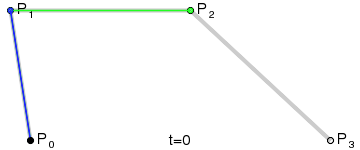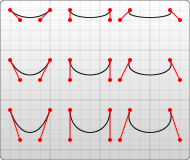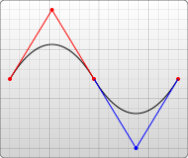html
``````<svg width="200" height="200">

<!-- 三次贝塞尔曲线 -->
<path d="M 10 10 C 20 20, 40 20, 50 10" />
<path d="M 70 10 C 70 20, 110 20, 110 10" />
<path d="M 130 10 C 120 20, 180 20, 170 10" />
<path d="M 10 60 C 20 80, 40 80, 50 60" />
<path d="M 70 60 C 70 80, 110 80, 110 60" />
<path d="M 130 60 C 120 80, 180 80, 170 60" />
<path d="M 10 110 C 20 140, 40 140, 50 110" />
<path d="M 70 110 C 70 140, 110 140, 110 110" />
<path d="M 130 110 C 120 140, 180 140, 170 110" />

<!-- 平滑三次贝塞尔曲线 -->
<path d="M 10 80 C 40 10, 65 10, 95 80 S 150 150, 180 80" />

<!-- 二次贝塞尔曲线 -->
<path d="M 10 80 Q 95 10 180 80" />

<!-- 平滑二次贝塞尔曲线 -->
<path d="M 10 80 Q 52.5 10, 95 80 T 180 80" />
</svg>
</div>

<!--
三次贝塞尔曲线，通过两个坐标点来控制曲线程度：
C：x1 y1, x2 y2, x y
x1 y1：起点控制点
x2 y2：终点控制点
x y：曲线终点

平滑三次贝塞尔曲线，在三次贝塞尔上加个对称：
S：x2 y2, x y
x2 y2：第一个控制点（若单独使用，就是当前点；若前面有C或Q，就是前面的结尾点的中心对称点）
x y：曲线终点

二次贝塞尔曲线，通过两个坐标点来控制曲线程度：
C：x1 y1, x y
x1 y1：起点控制点
x y：曲线终点

平滑二次贝塞尔曲线，通过一个坐标点来控制曲线程度，和平滑三次贝塞尔曲线同理：
T： x y
x y：曲线终点
-->
``````
``````<svg width="200" height="200">

<!-- 三次贝塞尔曲线 -->
<path d="M 10 10 C 20 20, 40 20, 50 10" />
<path d="M 70 10 C 70 20, 110 20, 110 10" />
<path d="M 130 10 C 120 20, 180 20, 170 10" />
<path d="M 10 60 C 20 80, 40 80, 50 60" />
<path d="M 70 60 C 70 80, 110 80, 110 60" />
<path d="M 130 60 C 120 80, 180 80, 170 60" />
<path d="M 10 110 C 20 140, 40 140, 50 110" />
<path d="M 70 110 C 70 140, 110 140, 110 110" />
<path d="M 130 110 C 120 140, 180 140, 170 110" />

<!-- 平滑三次贝塞尔曲线 -->
<path d="M 10 80 C 40 10, 65 10, 95 80 S 150 150, 180 80" />

<!-- 二次贝塞尔曲线 -->
<path d="M 10 80 Q 95 10 180 80" />

<!-- 平滑二次贝塞尔曲线 -->
<path d="M 10 80 Q 52.5 10, 95 80 T 180 80" />
</svg>
</div>

<!--
三次贝塞尔曲线，通过两个坐标点来控制曲线程度：
C：x1 y1, x2 y2, x y
x1 y1：起点控制点
x2 y2：终点控制点
x y：曲线终点

平滑三次贝塞尔曲线，在三次贝塞尔上加个对称：
S：x2 y2, x y
x2 y2：第一个控制点（若单独使用，就是当前点；若前面有C或Q，就是前面的结尾点的中心对称点）
x y：曲线终点

二次贝塞尔曲线，通过两个坐标点来控制曲线程度：
C：x1 y1, x y
x1 y1：起点控制点
x y：曲线终点

平滑二次贝塞尔曲线，通过一个坐标点来控制曲线程度，和平滑三次贝塞尔曲线同理：
T： x y
x y：曲线终点
-->
``````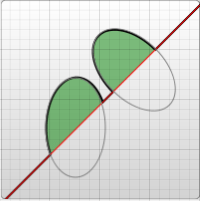html
``````<svg width="320" height="320">

<!-- 图1 -->
<path
d="M 10 315
L 110 215
A 30 50 0 0 1 162.55 162.45
L 172.55 152.45
A 30 50 -45 0 1 215.1 109.9
L 315 10"
stroke="black" fill="green" stroke-width="2" fill-opacity="0.5" />

<!-- 图2 -->
<path
d="M 10 315
L 110 215
A 36 60 0 0 1 150.71 170.29
L 172.55 152.45
A 30 50 -45 0 1 215.1 109.9
L 315 10"
stroke="black" fill="green" stroke-width="2" fill-opacity="0.5" />
<circle cx="150.71" cy="170.29" r="2" fill="red" />
<circle cx="110" cy="215" r="2" fill="red" />
<ellipse cx="144.931" cy="229.512" rx="36" ry="60" fill="transparent" stroke="blue" />
<ellipse cx="115.779" cy="155.778" rx="36" ry="60" fill="transparent" stroke="blue" />
</svg>

<!--
弧线，可参考椭圆的参数理解：
A：rx ry x-axis-rotation large-arc-flag sweep-flag x y
rx ry：x轴和y轴半径
x-axis-rotation：x轴旋转角度
large-arc-flag：角度大小
sweep-flag：弧线方向
x y：弧线终点
-->
``````
``````<svg width="320" height="320">

<!-- 图1 -->
<path
d="M 10 315
L 110 215
A 30 50 0 0 1 162.55 162.45
L 172.55 152.45
A 30 50 -45 0 1 215.1 109.9
L 315 10"
stroke="black" fill="green" stroke-width="2" fill-opacity="0.5" />

<!-- 图2 -->
<path
d="M 10 315
L 110 215
A 36 60 0 0 1 150.71 170.29
L 172.55 152.45
A 30 50 -45 0 1 215.1 109.9
L 315 10"
stroke="black" fill="green" stroke-width="2" fill-opacity="0.5" />
<circle cx="150.71" cy="170.29" r="2" fill="red" />
<circle cx="110" cy="215" r="2" fill="red" />
<ellipse cx="144.931" cy="229.512" rx="36" ry="60" fill="transparent" stroke="blue" />
<ellipse cx="115.779" cy="155.778" rx="36" ry="60" fill="transparent" stroke="blue" />
</svg>

<!--
弧线，可参考椭圆的参数理解：
A：rx ry x-axis-rotation large-arc-flag sweep-flag x y
rx ry：x轴和y轴半径
x-axis-rotation：x轴旋转角度
large-arc-flag：角度大小
sweep-flag：弧线方向
x y：弧线终点
-->
``````

`large-arc-flag` 决定弧线是大于还是小于 180 度，0 表示小角度弧，1 表示大角度弧。`sweep-flag` 表示弧线的方向，0 表示从起点到终点沿逆时针画弧，1 表示从起点到终点沿顺时针画弧。下面是一个例子：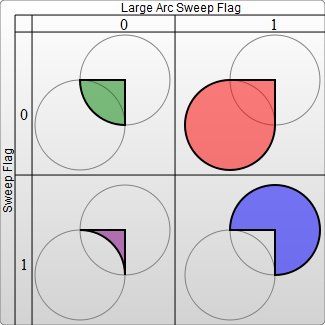html
``````<svg width="325" height="325">
<path
d="M 80 80 A 45 45, 0, 0, 0, 125 125 L 125 80 Z"
fill="green"
/>
<path
d="M 230 80 A 45 45, 0, 1, 0, 275 125 L 275 80 Z"
fill="red"
/>
<path
d="M 80 230 A 45 45, 0, 0, 1, 125 275 L 125 230 Z"
fill="purple"
/>
<path d="M 230 230 A 45 45, 0, 1, 1, 275 275 L 275 230 Z"
fill="blue"
/>
</svg>
``````
``````<svg width="325" height="325">
<path
d="M 80 80 A 45 45, 0, 0, 0, 125 125 L 125 80 Z"
fill="green"
/>
<path
d="M 230 80 A 45 45, 0, 1, 0, 275 125 L 275 80 Z"
fill="red"
/>
<path
d="M 80 230 A 45 45, 0, 0, 1, 125 275 L 125 230 Z"
fill="purple"
/>
<path d="M 230 230 A 45 45, 0, 1, 1, 275 275 L 275 230 Z"
fill="blue"
/>
</svg>
``````

### 填充 #

• `fill-opacity` 表示填充的不透明度。
• `stroke-opacity` 表示边框的不透明度。
• `stroke-linecap` 表示描边方式：`butt` | `square` | `round`
• `stroke-linejoin` 表示边框终点样式：`miter` | `bevel` | `round`
• `stroke-dasharray` 表示边框虚线宽度，每个数字都代表宽度（缝隙也算），然后循环。

stroke-linecap

stroke-linejoin

stroke-dasharray

html
``````<svg width="160" height="120">
<line
x1="80" x2="120" y1="20" y2="20" stroke="gray" stroke-width="20"
stroke-linecap="butt"/>
<line
x1="80" x2="120" y1="60" y2="60" stroke="gray" stroke-width="20"
stroke-linecap="square"/>
<line
x1="80"x2="120"y1="100"y2="100"stroke="gray" stroke-width="20"
stroke-linecap="round"/>

<polyline points="80 40 100 20 120 40" stroke="gray" stroke-width="10"
stroke-linecap="butt" stroke-linejoin="miter"/>
<polyline points="80 70 100 50 120 70" stroke="gray" stroke-width="10"
stroke-linecap="round" stroke-linejoin="round"/>
<polyline points="80 100 100 80 120 100" stroke="gray" stroke-width="10"
stroke-linecap="square" stroke-linejoin="bevel"/>

<path d="M80 8  L 160 8" stroke="gray" stroke-width="4"
stroke-dasharray="5,5" />
<path d="M80 55 L 160 55" stroke="gray" stroke-width="4"
stroke-dasharray="10,10" />
<path d="M80 100 L 160 100" stroke="gray" stroke-width="4"
stroke-dasharray="20,5,10" />
</svg>

``````
``````<svg width="160" height="120">
<line
x1="80" x2="120" y1="20" y2="20" stroke="gray" stroke-width="20"
stroke-linecap="butt"/>
<line
x1="80" x2="120" y1="60" y2="60" stroke="gray" stroke-width="20"
stroke-linecap="square"/>
<line
x1="80"x2="120"y1="100"y2="100"stroke="gray" stroke-width="20"
stroke-linecap="round"/>

<polyline points="80 40 100 20 120 40" stroke="gray" stroke-width="10"
stroke-linecap="butt" stroke-linejoin="miter"/>
<polyline points="80 70 100 50 120 70" stroke="gray" stroke-width="10"
stroke-linecap="round" stroke-linejoin="round"/>
<polyline points="80 100 100 80 120 100" stroke="gray" stroke-width="10"
stroke-linecap="square" stroke-linejoin="bevel"/>

<path d="M80 8  L 160 8" stroke="gray" stroke-width="4"
stroke-dasharray="5,5" />
<path d="M80 55 L 160 55" stroke="gray" stroke-width="4"
stroke-dasharray="10,10" />
<path d="M80 100 L 160 100" stroke="gray" stroke-width="4"
stroke-dasharray="20,5,10" />
</svg>

``````

### 渐变 #

SVG 可以做到 线性渐变径向渐变，不过你得用一个 `id` 属性让它去识别，得用一个 `<defs>` 标签来包裹渐变的内容，得用一个 `<linearGradient>` 标签来包裹线性的渐变方式，得用一个 `<radialGradient>` 标签来包裹径向的渐变方式。

#### 线性渐变 #

html
``````<svg width="480" height="120">

<!-- 用 defs 包裹，linearGradient 设定渐变内容 -->
<defs>
<stop offset="0%" stop-color="violet"/>
<stop offset="40%" stop-color="orange" stop-opacity="0.6"/>
<stop offset="100%" stop-color="green"/>
<stop offset="0%" stop-color="cyan"/>
<stop offset="100%" stop-color="pink"/>
</defs>

<rect
x="0" y="10" rx="15" ry="15" width="100" height="100"
/>
<rect
x="120" y="10" rx="15" ry="15" width="100" height="100"
/>
</svg>

<!--
x1 y1 x2 y2：表示水平/垂直方向的渐变，
第一个为 x 不变，y 递进，
第二个为 y 不变，x 递进。
-->
``````
``````<svg width="480" height="120">

<!-- 用 defs 包裹，linearGradient 设定渐变内容 -->
<defs>
<stop offset="0%" stop-color="violet"/>
<stop offset="40%" stop-color="orange" stop-opacity="0.6"/>
<stop offset="100%" stop-color="green"/>
<stop offset="0%" stop-color="cyan"/>
<stop offset="100%" stop-color="pink"/>
</defs>

<rect
x="0" y="10" rx="15" ry="15" width="100" height="100"
/>
<rect
x="120" y="10" rx="15" ry="15" width="100" height="100"
/>
</svg>

<!--
x1 y1 x2 y2：表示水平/垂直方向的渐变，
第一个为 x 不变，y 递进，
第二个为 y 不变，x 递进。
-->
``````

#### 径向渐变 #

html
``````<svg width="500" height="120">
<defs>
<stop offset="40%" stop-color="orange" />
<stop offset="100%" stop-color="teal"/>
<stop offset="0%" stop-color="yellow"/>
<stop offset="100%" stop-color="lightblue"/>
<stop offset="0%" stop-color="cyan"/>
<stop offset="100%" stop-color="pink"/>
</defs>
<rect
x="0" y="10" rx="15" ry="15" width="100" height="100"
/>
<rect
x="200" y="10" rx="15" ry="15" width="100" height="100"
/>
<rect
x="400" y="10" rx="15" ry="15" width="100" height="100"
/>
</svg>

<!--
fx fy：焦点，表示渐变的中心，
cx cy r：渐变的边缘位置（圆环），r为半径
-->
``````
``````<svg width="500" height="120">
<defs>
<stop offset="40%" stop-color="orange" />
<stop offset="100%" stop-color="teal"/>
<stop offset="0%" stop-color="yellow"/>
<stop offset="100%" stop-color="lightblue"/>
<stop offset="0%" stop-color="cyan"/>
<stop offset="100%" stop-color="pink"/>
</defs>
<rect
x="0" y="10" rx="15" ry="15" width="100" height="100"
/>
<rect
x="200" y="10" rx="15" ry="15" width="100" height="100"
/>
<rect
x="400" y="10" rx="15" ry="15" width="100" height="100"
/>
</svg>

<!--
fx fy：焦点，表示渐变的中心，
cx cy r：渐变的边缘位置（圆环），r为半径
-->
``````

### 图案 #

`图案（Pattern）` 的使用方式和渐变很类似，不过它可以重复：

html
``````<svg width="200" height="200">
<defs>
<stop offset="5%" stop-color="white"/>
<stop offset="5%" stop-color="burlywood"/>
<stop offset="95%" stop-color="sandybrown"/>
<pattern id="Pattern" x="0" y="0" width=".25" height=".25">
<rect x="0" y="0" width="50" height="50" fill="skyblue"/>
<rect x="0" y="0" width="25" height="25" fill="url(#Gradient8)"/>
</pattern>
</defs>
<rect fill="url(#Pattern)" x="0" y="0" width="200" height="200"/>
</svg>

<!--
描述：一个矩形用长宽比为 25% 的 pattern 重复填充（会填充 4 次），在 pattern 里有矩形1、
pattern 中的 x 和 y 可以定义偏移的开始点。
-->
``````
``````<svg width="200" height="200">
<defs>
<stop offset="5%" stop-color="white"/>
<stop offset="5%" stop-color="burlywood"/>
<stop offset="95%" stop-color="sandybrown"/>
<pattern id="Pattern" x="0" y="0" width=".25" height=".25">
<rect x="0" y="0" width="50" height="50" fill="skyblue"/>
<rect x="0" y="0" width="25" height="25" fill="url(#Gradient8)"/>
</pattern>
</defs>
<rect fill="url(#Pattern)" x="0" y="0" width="200" height="200"/>
</svg>

<!--
描述：一个矩形用长宽比为 25% 的 pattern 重复填充（会填充 4 次），在 pattern 里有矩形1、
pattern 中的 x 和 y 可以定义偏移的开始点。
-->
``````

### 文字 #

html
``````<svg width="420" height="200">
<text x="10" y="110" fill="orange" font-size="25">Love 💕</text>
<text
x="210 240 270 300 330 360 390"
y="50 80 110 140 110 80 50"
fill="orange"
font-size="25"
font-weight="bold"
>
evolove
</text>
</svg>

<!--
x y：可以是一个坐标，也可以是一个点集数列
-->
``````
``````<svg width="420" height="200">
<text x="10" y="110" fill="orange" font-size="25">Love 💕</text>
<text
x="210 240 270 300 330 360 390"
y="50 80 110 140 110 80 50"
fill="orange"
font-size="25"
font-weight="bold"
>
evolove
</text>
</svg>

<!--
x y：可以是一个坐标，也可以是一个点集数列
-->
``````

`rotate` 可以旋转文字，用法分为整体旋转角度和单个文字旋转角度：

html
``````<svg width="120" height="70">
<text x="30" y="20" fill="orange" font-size="20" rotate="20">
e v o l o v e
</text>
<!--
<text x="150" y="50" fill="orange" font-size="20" rotate="0 20 40 60 80 100">
e v o l o v e
</text>
-->
</svg>
``````
``````<svg width="120" height="70">
<text x="30" y="20" fill="orange" font-size="20" rotate="20">
e v o l o v e
</text>
<!--
<text x="150" y="50" fill="orange" font-size="20" rotate="0 20 40 60 80 100">
e v o l o v e
</text>
-->
</svg>
``````

html
``````<svg width="350" height="130">
<text x="50" y="50" textLength="150" fill="orange" font-size="20">
Hello World !
</text>
<text  x="50" y="100" textLength="300" fill="orange"  font-size="20">
Hello World !
</text>
</svg>
``````
``````<svg width="350" height="130">
<text x="50" y="50" textLength="150" fill="orange" font-size="20">
Hello World !
</text>
<text  x="50" y="100" textLength="300" fill="orange"  font-size="20">
Hello World !
</text>
</svg>
``````

### 裁剪和遮罩 #

html
``````<svg width="300" height="300">
<defs>
<clipPath id="clipPath">
<path d="M10 50 A50 50 0 0 1 100 50 A50 50 0 0 1 190 50 Q210 100 100 200  Q-5 100 10 50 Z" />
</clipPath>
</defs>
<rect x="0" y="0" width="200" height="200" fill="orange" clip-path="url(#clipPath)"  />
</svg>

<!--
描述：在一个矩形里，裁剪出一个心形
-->
``````
``````<svg width="300" height="300">
<defs>
<clipPath id="clipPath">
<path d="M10 50 A50 50 0 0 1 100 50 A50 50 0 0 1 190 50 Q210 100 100 200  Q-5 100 10 50 Z" />
</clipPath>
</defs>
<rect x="0" y="0" width="200" height="200" fill="orange" clip-path="url(#clipPath)"  />
</svg>

<!--
描述：在一个矩形里，裁剪出一个心形
-->
``````

html
``````<svg>
<defs>
<stop offset="0" stop-color="white" stop-opacity="0" />
<stop offset="1" stop-color="white" stop-opacity="1" />
<rect x="0" y="0" width="200" height="200" fill="url(#Gradient10)"  />
</defs>
<rect x="0" y="0" width="200" height="200" fill="orange" />
</svg>

<!--
描述：底色是橘色，顶色是青色，过度到青色的时候用一个白色的遮罩进行过度，
而遮罩本身用了一个渐变色。
-->
``````
``````<svg>
<defs>
<stop offset="0" stop-color="white" stop-opacity="0" />
<stop offset="1" stop-color="white" stop-opacity="1" />
<rect x="0" y="0" width="200" height="200" fill="url(#Gradient10)"  />
</defs>
<rect x="0" y="0" width="200" height="200" fill="orange" />
</svg>

<!--
描述：底色是橘色，顶色是青色，过度到青色的时候用一个白色的遮罩进行过度，
而遮罩本身用了一个渐变色。
-->
``````